Home > AC > Chapter 11 > Lesson 11.1.5 > Problem11-62

11-62.
1.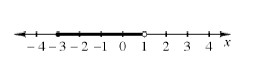One way to represent the solutions shown on the number line at right is −3 < x < 1. For each number line below, write a similar mathematical sentence to describe the solutions for x. Homework Help ✎

1.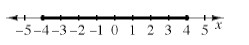2.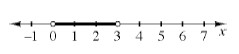3.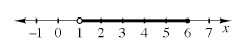4.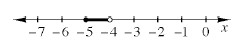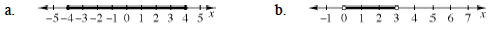What is the lowest number x can be?

Does the graph include this number?

What is the highest number x can be?

Does the graph include this number?

−4 ≤ x ≤ 4

Follow the steps in part (a).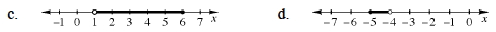Follow the steps in part (a).

Follow the steps in part (a).